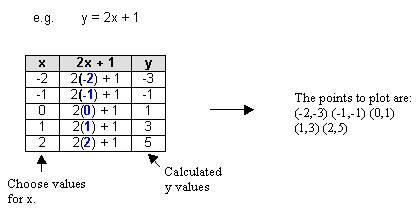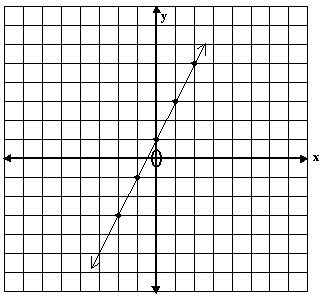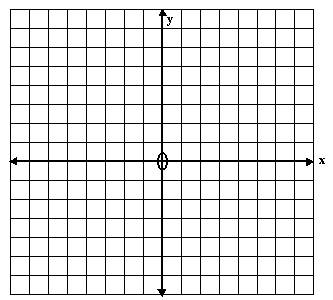# Drawing Straight Line Graphs Tutorial

math tutorials > drawing straight line graphs from a formula

How to draw a straight line graph from an equation.

## Straight Line Graph Equations

The general equation for a straight line is:  y = mx + c

• x and y have a power of 1
• m is the gradient (steepness) of the line and is a number
• c is the y-intercept of the line and is a number.

## Examples

These are all straight line graphs:

• y = 2x + 1
• y = x
• y = 7
• x = -2
• 3y + x = 1

## Drawing lines using the tabular method

The graph is drawn by setting up a table of values for x and calculating the corresponding y value using the equation given.  The points are then plotted and joined by a straight line.## Graphing problems for you to try

1)  y = 3x + 2
2)  x + y = 5
3)  y = 4 – x